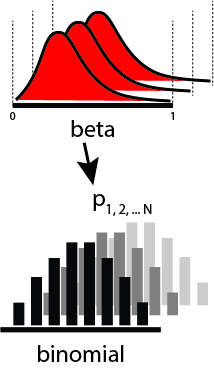# Introduction

This notebook shows how to do hierarchical modelling with Binomially-distributed random variables.

## Problem Setup

Baseball players have many metrics measured for them. Let's say we are on a baseball team, and would like to quantify player performance, one metric being their batting average (defined by how many times a batter hit a pitched ball, divided by the number of times they were up for batting ("at bat")). How would you go about this task?

We first need some measurements of batting data. To answer this question, we need to have data on the number of time a player has batted and the number of times the player has hit the ball while batting. Let's see an example dataset below.

``````%load_ext autoreload
%matplotlib inline
%config InlineBackend.figure_format = 'retina'
``````
``````import pandas as pd
import pymc3 as pm
import matplotlib.pyplot as plt
import numpy as np
import theano.tensor as tt
from pyprojroot import here
``````
``````WARNING (theano.configdefaults): install mkl with `conda install mkl-service`: No module named 'mkl'
```
```
``````df = pd.read_csv(here() / "datasets/baseballdb/core/Batting.csv")
df["AB"] = df["AB"].replace(0, np.nan)
df = df.dropna()
df["batting_avg"] = df["H"] / df["AB"]
df = df[df["yearID"] >= 2016]
df = df.iloc[
0:15
]  # select out only the first 15 players, just for illustration purposes.
``````
playerID yearID stint teamID lgID G AB R H 2B ... SB CS BB SO IBB HBP SH SF GIDP batting_avg
101333 abadfe01 2016 1 MIN AL 39 1.0 0 0 0 ... 0.0 0.0 0 1.0 0.0 0.0 0.0 0.0 0.0 0.000000
101335 abreujo02 2016 1 CHA AL 159 624.0 67 183 32 ... 0.0 2.0 47 125.0 7.0 15.0 0.0 9.0 21.0 0.293269
101337 ackledu01 2016 1 NYA AL 28 61.0 6 9 0 ... 0.0 0.0 8 9.0 0.0 0.0 0.0 1.0 0.0 0.147541
101338 adamecr01 2016 1 COL NL 121 225.0 25 49 7 ... 2.0 3.0 24 47.0 0.0 4.0 3.0 0.0 5.0 0.217778
101340 adamsma01 2016 1 SLN NL 118 297.0 37 74 18 ... 0.0 1.0 25 81.0 1.0 2.0 0.0 3.0 5.0 0.249158

5 rows × 23 columns

In this dataset, the columns `AB` and `H` are the most relevent.

• `AB` is the number of times a player was At Bat.
• `H` is the number of times a player hit the ball while batting.

The performance of a player can be defined by their batting percentage - essentially the number of hits divided by the number of times at bat. (Technically, a percentage should run from 0-100, but American sportspeople are apparently not very strict with how they approach these definitions.)

## Model 1: Naive Model

One model that we can write is a model that assumes that each player has a batting percentage that is independent of the other players in the dataset.

A pictorial view of the model is as such:Let's implement this model in PyMC3.

``````with pm.Model() as baseline_model:
thetas = pm.Beta("thetas", alpha=0.5, beta=0.5, shape=(len(df)))
like = pm.Binomial("likelihood", n=df["AB"], p=thetas, observed=df["H"])
``````
``````with baseline_model:
baseline_trace = pm.sample(2000)
``````
``````Auto-assigning NUTS sampler...
Multiprocess sampling (4 chains in 4 jobs)
NUTS: [thetas]
Sampling 4 chains, 16 divergences: 100%|██████████| 10000/10000 [00:03<00:00, 2887.97draws/s]
There were 5 divergences after tuning. Increase `target_accept` or reparameterize.
There were 2 divergences after tuning. Increase `target_accept` or reparameterize.
There were 8 divergences after tuning. Increase `target_accept` or reparameterize.
There was 1 divergence after tuning. Increase `target_accept` or reparameterize.
```
```

Let's view the posterior distribution traces.

``````import arviz as az

traceplot = az.plot_trace(baseline_trace)
``````